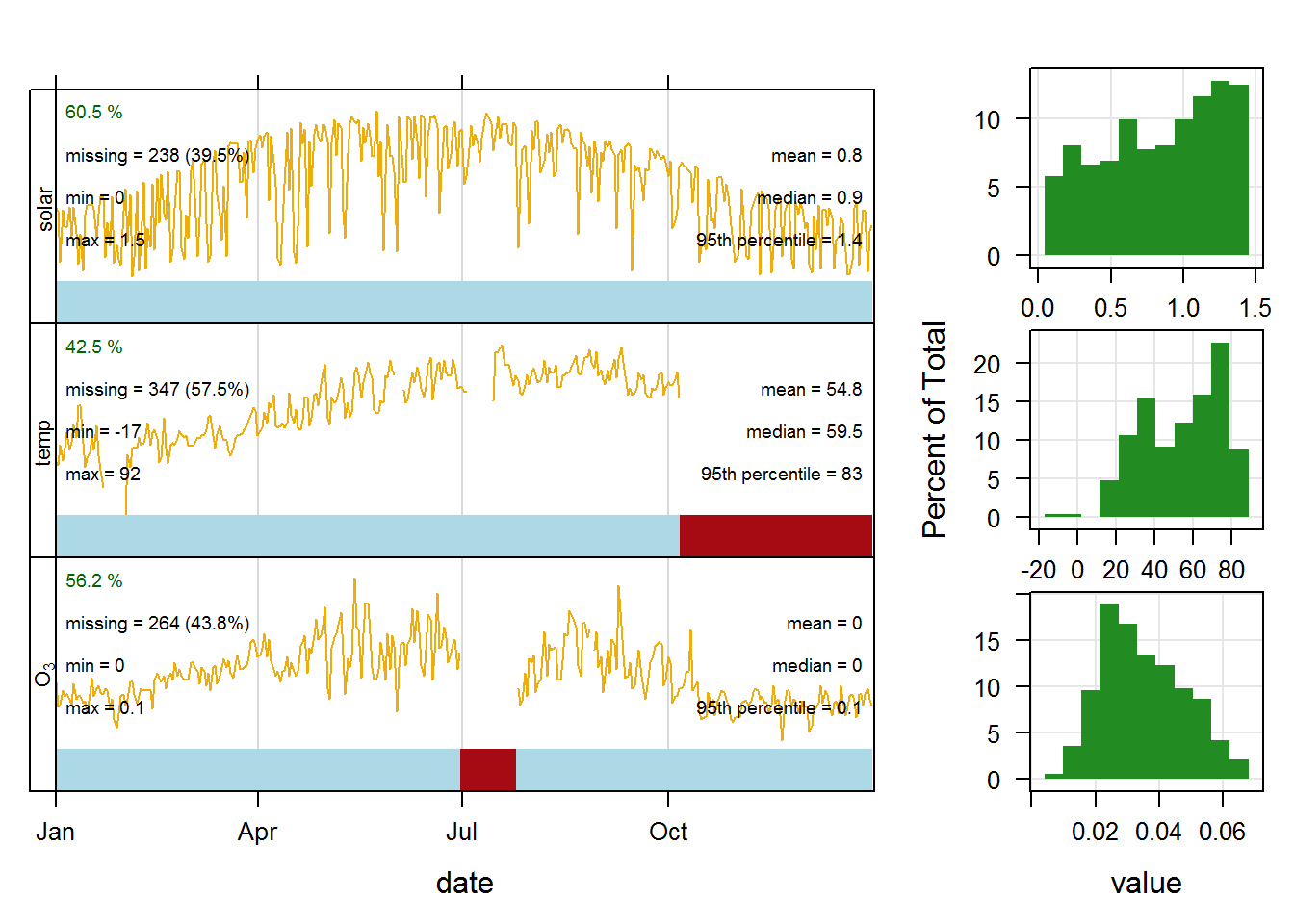The `openair` package is specifically designed to plot air pollution data. This tutorial will give a brief introduction to many of the plotting functions in `openair`. Visit the project website for more information, including a comprehensive manual.

This tutorial will cover the following `openair` functions.

## `summaryPlot()`

The first plotting function we’ll look at is `summaryPlot()`. Functions in the `openair` package expect data frames that are in a certain format. The column with date and time information must be labeled “date” (lowercase) and be a `POSIXct` class.

The first data frame we’ll use is the `chicago_air` data frame from the `region5air` package, which already has a column labeled “date”.

``````library(region5air)
data(chicago_air)
``````##         date ozone temp solar month weekday
## 1 2013-01-01 0.032   17  0.65     1       3
## 2 2013-01-02 0.020   15  0.61     1       4
## 3 2013-01-03 0.021   28  0.17     1       5
## 4 2013-01-04 0.028   18  0.62     1       6
## 5 2013-01-05 0.025   26  0.48     1       7
## 6 2013-01-06 0.026   36  0.47     1       1``````

However, the class of that “date” column is `character`.

``class(chicago_air\$date)``
``##  "character"``

We need to change it using the `as.POSIXct()` function. This function will assume that the format of the date is YYYY-MM-DD. So we just need to supply a correct time zone.

``chicago_air\$date <- as.POSIXct(chicago_air\$date, tz = "America/Chicago")``

If your date (and time) isn’t in the default format, see the “Working with Dates in R” section of this tutorial. If you need to provide a different time zone, run the `OlsonNames()` function for a list of options.

Now we just feed the first four columns of the data frame to the `summaryPlot()` function. We use the `select()` function from `dplyr` to select our columns, and we use the short hand `date:solar` to select the “date” column, the “solar” column, and all the columns in between.

``````library(openair)
library(dplyr)

summaryPlot(select(chicago_air, date:solar))``````
``````##     date1     date2     ozone      temp     solar
## "POSIXct"  "POSIXt" "numeric" "numeric" "numeric"``````The first column of the graph contains time series plots of all of the parameters in the data frame. The red bars at the bottom of each panel show where there are large segments of missing data. The panels on the right are the histograms of the distributions for each parameter.

Note: If the plot does not look correct, there may be an issue with the date formatting. Use the code below to save the data to a .csv file, read it back into R using the `import()` function from `openair`, and plot the imported data.

``````# save a .csv file of the data to your working directory
write.csv(chicago_air, file = "chicago_air.csv", row.names = FALSE)

# import the data
chicago_air <- import(file = "chicago_air.csv", date.format = "%Y-%m-%d",
tzone = "America/Chicago")``````
``````##     date1     date2     ozone      temp     solar     month   weekday
## "POSIXct"  "POSIXt" "numeric" "integer" "numeric" "integer" "integer"``````
``summaryPlot(select(chicago_air, date:solar))``
``````##     date1     date2     ozone      temp     solar
## "POSIXct"  "POSIXt" "numeric" "integer" "numeric"``````## `windRose()`

The `windRose()` function expects a data frame with columns for wind speed and wind direction labeled “ws” and “wd”, respectively. Here we load the `chicago_wind` dataset from the `region5air` package and take a look at the columns.

``````data(chicago_wind)
``````## Source: local data frame [6 x 4]
## Groups: datetime 
##
##             datetime wind_speed wind_dir ozone
##                (chr)      (dbl)    (dbl) (dbl)
## 1 20130101T0000-0600        2.0      334    NA
## 2 20130101T0100-0600        2.0      321    NA
## 3 20130101T0200-0600        2.0      323    NA
## 4 20130101T0300-0600        1.6      324    NA
## 5 20130101T0400-0600        1.6      319    NA
## 6 20130101T0500-0600        1.4      319    NA``````

We need to create a “date” column with a `POSIXct` class, so we’ll use the `as.POSIXct()` function again. This time we will need to provide information in the `format` parameter.

``````chicago_wind\$datetime <- as.POSIXct(chicago_wind\$datetime, format ="%Y%m%dT%H%M",
tz = "America/Chicago")``````

Now we’ll rename the columns using the `rename()` function from `dplyr`.

``chicago_wind <- rename(chicago_wind, date = datetime, ws = wind_speed, wd = wind_dir)``

Note: You can also import data directly into openair using the `import()` function. This will accept .csv and .txt files. The `import()` function is just a wrapper for `read.table` that has been customized for use in `openair`. The code below is intended for example only.

``````#           oz.data <- import('C:/mydata/myozonedata.csv', na.strings = c('-99'',-999'),
#                            date = 'mydate', date.format = '%Y-%m-%d %H:%M',
#                             ws = 'wind.speed', wd = 'wind.dir')``````

Now back to our `chicago_wind` data frame. We have prepared the dates and column names above so that now we can feed the data frame to the `windRose()` function.

``windRose(chicago_wind, key.footer = "knots") # default is m/s``